# Distribution Post Processing

Analyze distributions of run data.

## Analyze Distributions of Run Data

Analyze all the distributions of run data in a histogram or box plot from the Scatter post processing tab.

1. From the Post Processing step, click the Distribution tab.
2. From the Channel selector, select the channels to plot.
3. Switch the view between histogram and box plot by clicking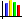or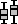, located above the Channel selector.
Tip: Display selected data in a single plot or separate plots by switching the Multiplot option between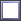(single plot) and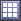(multiple plots).
Configure the plot's display settings by clicking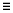(located above the Channel selector). For more information about these settings, refer to Distribution Tab Settings.

## Distribution Tab Settings

Settings to configure the plots displayed in the Distribution post processing tab.

Access settings for the histogram from the menu that displays when you click(located above the Channel selector).
Histogram
Turn the display of histogram bins on and off.
Probability density (PDF)
Turn the display of PDF curves on and off.
Cumulative distribution (CDF)
Turn the display of CDF curves on and off.
Bins
Change the number of bins that displays.

A box plot sorts data and draws a box from the lower quartile (1st quartile, Q1, 25%) to the upper quartile (3rd quartile, Q3, 75%).

Quartiles of a sorted data set consist of the three points (Q1, Q2 which is also the median, and Q3) that divide the data set into four groups, each group comprising a quarter of the data. The median and mean of the data are also marked in the box. In HyperStudy, this box is painted dark green.

Box plots may also have lines extending vertically from the box to indicate the data outside the lower and upper quartiles. Furthermore, to identify outliers, these lines may extend only to the whiskers as opposed to the minimum and maximum of the data. Whisker location is calculated as a function of lower and upper quartile and the difference between them (this difference is known as interquartile range, IQR) as:
Lower whisker
Q1 – 1.5*IQR
Upper whisker
Q3 + 1.5*IQ
Any data that is not within the whiskers are identified as outliers. In HyperStudy, whiskers are displayed as a light green box instead of as a vertical line, and data points are indicated by blue dots. Horizontal scale is their run number and vertical scale is their value.

Box plots display the distribution of data. Use box plots to find the range, mean, median, quartiles, whiskers and outliers. This information tells you the spread and skewness of the data and helps you identify outliers. It is important that you understand the spread and skewness in order to understand and improve the variations in the data. Identifying the outliers gives you an opportunity to investigate these data points and resolve possible issues that you may have missed.

Figure 2 is a comparison of a box plot of data sampled from a normal distribution to the theoretical probability distribution function of the normal distribution. The dark green color indicates the interquartile range, the light green color indicates the range of the whiskers, and the red color indicates outliers.